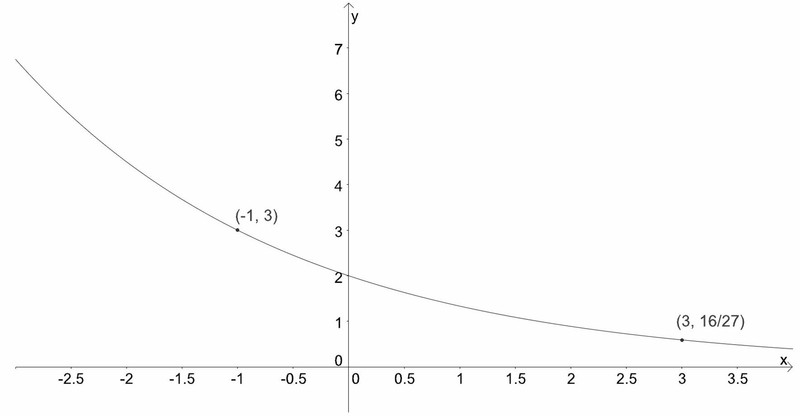# Two Points Determine an Exponential Function II

Alignments to Content Standards: F-LE.A.2

The graph of a function of the form $f(x)=ab^x$ is shown below. Find the values of $a$ and $b$.## IM Commentary

An easier version of this problem is given in Two Points Determine an Exponential Function, I

## Solution

The value of the function decreases from 3 to $\frac{16}{27}$ by multiplying 3 four times by $b$ $$3 \times b \times b \times b \times b = \frac{16}{27}$$

Writing this as $3b^4 = \frac{16}{27}$, we divide by $3$ to get $b^4 = \frac{16}{81}$, so $b=\pm \frac{2}{3}$  Since the base $b$ must be positive, we conclude that $b = \frac{2}{3}$.

Now, since $(-1,3)$ is on the graph of $f$, we know $f(-1)=3$, so substituting this input-output pair into the equation yields
\begin{align} 3=&a\left(\frac{2}{3}\right)^{-1},\end{align} which quickly leads to $a=2$.  We conclude that $f(x) = 2\left(\frac{2}{3}\right)^x$